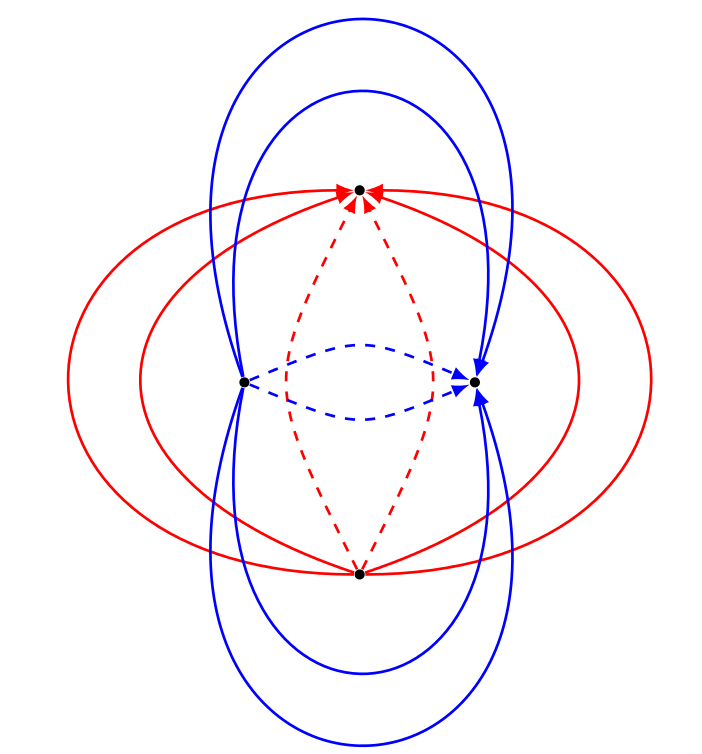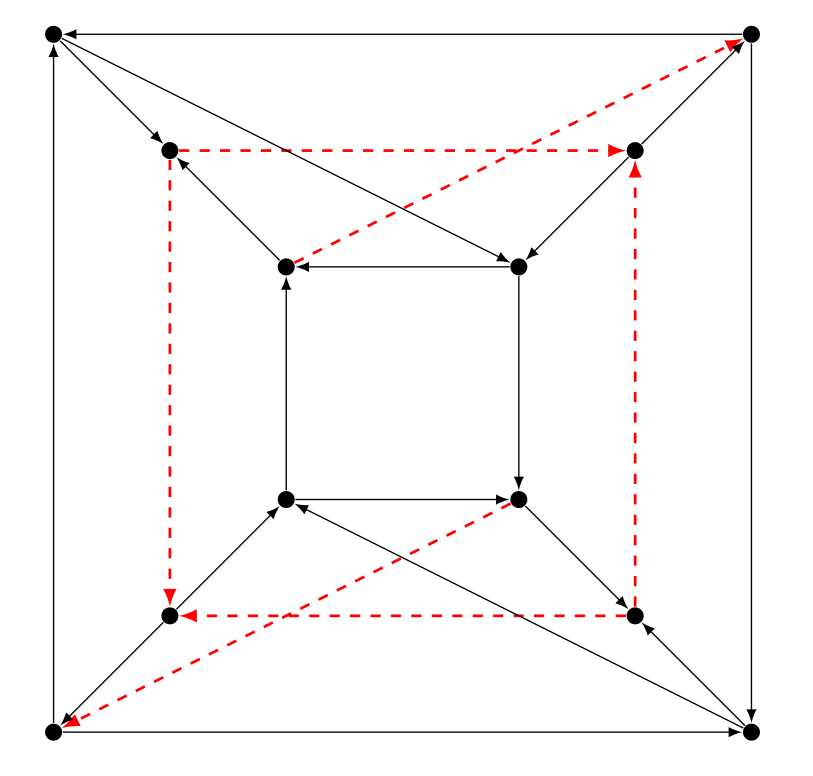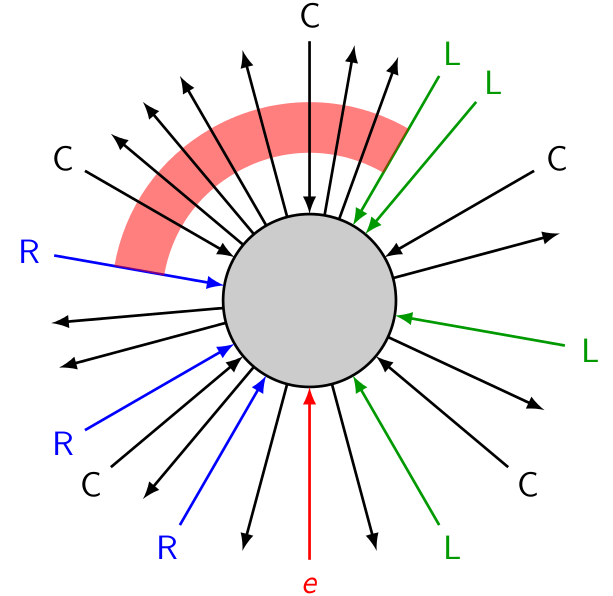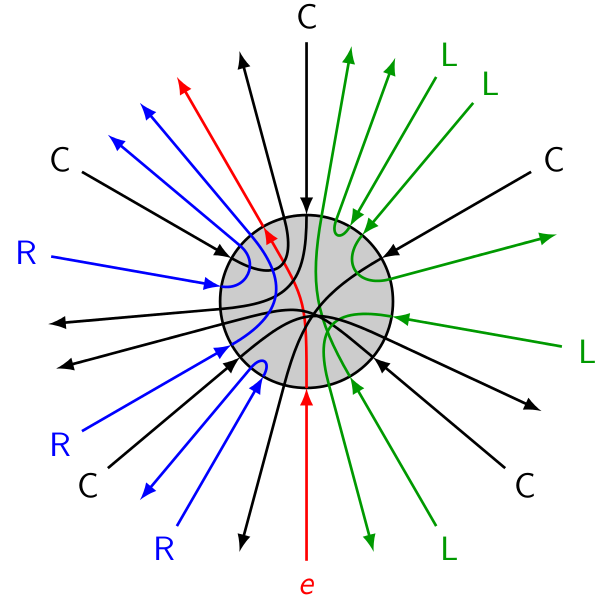## Routing in planar dags with Eulerian condition

When dealing with arc-disjoint paths problems, many hypothesis can make the problem easier. Usually one is not enough though, so we have to combine them if we want to find a polynomiality result. Here are a few interesting cases:

• the supply graph $G$ is planar,
• the supply graph is acyclic,
• all the capacities are $1$,
• the number of terminals, or the number of demand arcs, is bounded by a constant,
• the graph obtained by taking both the supply arcs and the demand arcs, which we call $G+H$, is Eulerian. By this, we mean that the sum of the capacities and demands entering any vertex is equal to the sum of capacities and demands leaving this vertex.

It was proven by Frank Pfeiffer and independantly by Dániel Marx that the arc-disjoint paths problem is still np-complete when we take all this additional assumption, except the one bounding the number of demands. One the other hand, with only three demand arcs with demand $1$, the Euler condition is sufficient to get a planar algorithm. Between these two results, we do not know a lot, the complexity of most of the possible combinations is open.

I gave a polynomial algorithm for the edge-disjoint paths problem when $G$ is planar and acyclic, $G+H$ eulerian and the number of demand arcs is fixed. That looks like very strong assumptions. Indeed, the solutions have very special structures.

Take a source $s$ of $G$, as $G$ is acyclic. Denote $c$ the total capacities leaving $s$ in $G$. Because $G$ is Eulerian, there must be demands in $H$ with a total request of $c$ too. Hence each arc leaving $s$ must be saturated in any solution.

Let's make this idea more general: take any directed cut $S$ in $G$. The capacity in $G$ leaving that cut must be equal to the request in $H$ going back through that cut (just sum the Euler condition on every vertex of $S$). So every arc of $C$ is saturated. Because $G$ is acyclic, all the arcs of $G$ are saturated.

We could hope that this makes the problem a lot easier. Let me show that this problem is still not completely trivial. For this, I will simply show a small graph with a fractional solution but no integral solution. This example is the smallest possible: with only five demands, the existence of a fractional solution implies the existence of an integral one. We proved this with Christophe Weibel by finding operations to reduce the graphs to a finite number of them, and then generating and checking them all. This is also how we found this example.Red dashed arcs correspond to demand arcs. The fractional solution consists in taking for each demand the two possible paths of length three with value a half. There is no optimal solution, I do not prove it, let's just say that the six demands have extemities at distance three, but there are 18 arcs, so it is sufficient to consider paths of length $3$.

Still, in the uncapacitated case, when $H$ contains only a fixed number of classes of parallel arcs, the problem can be solved polynomially. The proof is based on basic topological facts. Mainly, when considering two classes of parallel demands, their respective paths cannot cross each other in a completely arbitrary way. The idea is then to decide first how theses paths will cross each other. Using that information, we place an agent in every vertex, that is charged to decided for every path entering the vertex, where it must leave. Because of the forced relative behaviour of paths between each other, there is always at most one solution in each vertex. Therefore, once we have decided the crossing pattern, it is easy to check its feasibility. The algorithm follows from the fact that the number of possible patterns is small.In this picture, the red path must turn left compared to any green path, turn right compared to anyblue path, and it must cross every black path. You can check that in any such solution, if we don't change how the paths come into the node but only how they leave it, the red path leaves the node by the same leaving arc as in the solution below. This is how we ensure the uniqueness of the solution.